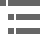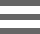## Depth of Field and Depth of FocusWhen considering resolution in optical microscopy, a majority of the emphasis is placed on point-to-point lateral resolution in the plane perpendicular to the optical axis (Figure 1). Another important aspect to resolution is the axial (or longitudinal) resolving power of an objective, which is measured parallel to the optical axis and is most often referred to as depth of field.

Axial resolution, like horizontal resolution, is determined only by the numerical aperture of the objective (Figure 2), with the eyepiece merely magnifying the details resolved and projected into the intermediate image plane. Just as in classical photography, depth of field is determined by the distance from the nearest object plane in focus to that of the farthest plane also simultaneously in focus. In microscopy depth of field is very short and usually measured in units of microns. The term depth of focus, which refers to image space, is often used interchangeably with depth of field, which refers to object space.

This interchange of nomenclature can lead to confusion, especially when the terms are both used specifically to denote depth of field in microscope objectives. The geometric image plane might be expected to represent an infinitely thin section of the specimen, but even in the absence of aberrations, each image point is spread into a diffraction figure that extends above and below this plane. The Airy disk, a basic unit of the diffraction pattern produced by the microscope objective, represents a section through the center of the intermediate image plane. This increases the effective in-focus depth of the Z-axis Airy disk intensity profile that passes through slightly different specimen planes.

Depth of focus varies with numerical aperture and magnification of the objective, and under some conditions, high numerical aperture systems (usually with higher magnification power) have deeper focus depths than do those systems of low numerical aperture, even though the depth of field is less (see Table 1). This is particularly important in photomicrography because the film emulsion or digital camera sensor must be exposed or illuminated in a plane that falls within the focus region. Small errors made to focus at high magnification are not as critical as those made with very low magnification objectives. Table 1 presents calculated variations in the depth of field and image depth in the intermediate image plane in a series of objectives with increasing numerical aperture and magnification.

At high numerical apertures of the microscope, depth of field is determined primarily by wave optics, while at lower numerical apertures, the geometrical optical circle of confusion dominates the phenomenon. Using a variety of different criteria for determining when the image becomes unacceptably sharp, several authors have proposed different formulas to describe the depth of field in a microscope. The total depth of field is given by the sum of the wave and geometrical optical depths of fields as:

Where d(tot) represents the depth of field, λ is the wavelength of illuminating light, n is the refractive index of the medium (usually air (1.000) or immersion oil (1.515)) between the coverslip and the objective front lens element, and NA equals the objective numerical aperture. The variable e is the smallest distance that can be resolved by a detector that is placed in the image plane of the microscope objective, whose lateral magnification is M. Using this equation, depth of field (d(tot)) and wavelength (λ) must be expressed in similar units. For example, if d(tot) is to be calculated in micrometers, λ must also be formulated in micrometers (700 nanometer red light is entered into the equation as 0.7 micrometers). Notice that the diffraction-limited depth of field (the first term in the equation) shrinks inversely with the square of the numerical aperture, while the lateral limit of resolution is reduced in a manner that is inversely proportional to the first power of the numerical aperture. Thus, the axial resolution and thickness of optical sections that can be attained are affected by the system numerical aperture much more so than is the lateral resolution of the microscope.

The human eye can normally accommodate from infinity to about 25 centimeters, so that the depth of field can considerably greater than that given by the equation above when one observes the microscope image through the eyepieces. On the other hand, a video sensor or photographic emulsion lies in a thin fixed plane so that the depth of field and axial resolution using those sensors are given by the parameters in the equation. In these cases, the axial resolution is defined by convention as one-quarter of the distance between the first minima, above and below focus, along the axis of the three-dimensional diffraction image produced by the objective.

These values for the depth of field, and the distribution of intensities in the three-dimensional diffraction pattern, are calculated for incoherently illuminated (or emitting) point sources where the numerical aperture of the condenser is greater than or equal to that of the objective. In general, the depth of field increases, up to a factor of 2, as the coherence of illumination increases (as the condenser numerical aperture approaches zero). However, the three-dimensional point spread function (PSF) with partially coherent illumination can depart in complex ways from that so far discussed when the aperture function is not uniform. In a number of phase-based, contrast-generating modes of microscopy, the depth of field may turn out to be unexpectedly shallower than that predicted from the equation above and may yield extremely thin optical sections.

In digital and video microscopy, the shallow focal plane in the target of the camera tube or CCD, the high contrast achievable at high objective and condenser numerical apertures, and the high magnification of the image displayed on the monitor all contribute to reducing the depth of field. Thus, with video, we can obtain very sharp and thin optical sections, and can define the focal level of a thin specimen with very high precision.

### Contributing Authors

Kenneth R. Spring - Scientific Consultant, Lusby, Maryland, 20657.

Michael W. Davidson - National High Magnetic Field Laboratory, 1800 East Paul Dirac Dr., The Florida State University, Tallahassee, Florida, 32310.

# Microscopy U - The source for microscopy education•••